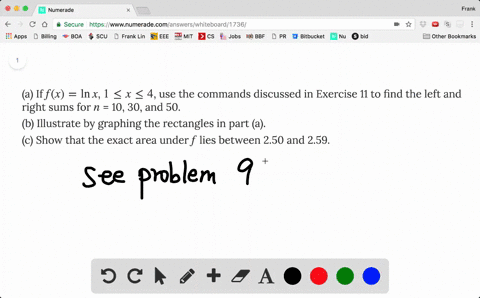### (a) If $f(x) = \ln x$, $1 \le x \le 4$, use t…

00:25
FL
Problem 11

Some computer algebra systems have commands that will draw approximating rectangles and evaluate the sums of their areas, at least if $x_{i}^{*}$ is a left or right endpoint. (For instance, in Maple use leftbox, rightbox, leftsum, and rightsum.)

(a) If $f(x) = 1/(x^2 + 1)$, $0 \le x \le 1$, find the left and right sums for $n$ = 10, 30, and 50.

(b) Illustrate by graphing the rectangles in part (a).

(c) Show that the exact area under $f$ lies between 0.780 and 0.791.

(A). $R_{50}=0.780381$
(B). Here's a picture of the graph
(C). 7.91

## Discussion

You must be signed in to discuss.

## Video Transcript

No transcript available